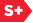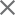### Proceedings Paper

Analysis on the Gaussian approximation of LP01 mode
Author(s): Lianhuang Li; Fuyuan Guo
Format Member Price Non-Member Price
PDF \$17.00 \$21.00

Paper Abstract

A new method that the equivalent mode field radius can be acquired according to the equivalent matching efficiency method is presented and the equation of the equivalent mode field radius, which is based on the core layer radius, the normalized standing wave parameter and the normalized evanescent wave parameter, is given. Moreover, adopted computer numerical simulation, a mathematical modeling between the equivalent mode field radius and the normalized frequency of fiber is established. Based on this, the equation between the equivalent mode field radius and the normalized frequency is introduced by curve fitting method. The numerical calculation indicates that it has a higher precision. As for weakly guiding optical fiber, referred to the characteristic of the beam propagation factor of diffractive beam of LP01 mode from its end surface, it was illuminated that the Gaussian approximation for the mode field distribution of LP01 mode is rational. The characteristic of the field of the Gaussian approximation, which is based on the equivalent mode field radius, is analyzed. The difference between the eigen mode field of LP01 mode and the field of its Gaussian approximation is discussed. From that, we can draw the conclusion that it is relatively accurate to adopt the equivalent matching efficiency method to obtain the equivalent mode field radius.

Paper Details

Date Published: 4 January 2008
PDF: 9 pages
Proc. SPIE 6837, Information Optics and Photonics Technologies II, 68370D (4 January 2008); doi: 10.1117/12.759646
Show Author Affiliations
Lianhuang Li, Fujian Normal Univ. (China)
Fuyuan Guo, Fujian Normal Univ. (China)

Published in SPIE Proceedings Vol. 6837:
Information Optics and Photonics Technologies II
Guoguang Mu; Feijun Song; Francis T. S. Yu; Suganda Jutamulia, Editor(s)